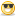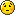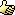CodeGuru Home VC++ / MFC / C++ .NET / C# Visual Basic VB Forums Developer.com

Thread: INT Function Rounding Problems

1.INT Function Rounding Problems

I have just spent the last few hours trying to find a problem where applying rounding after adding VAT was coming up with an odd few pence difference. Not that unusual you might think until I tripped over the code that was actually causing the porblem.

I have recreated the problem as an example as follows.....

Private Sub Command1_Click()
Dim TotVal As Currency
Dim VatRate As String

VatRate = "15"

TotVal = 2.1

MsgBox "Total VAT = " & ((TotVal * Val(VatRate)) + 0.5) / 100
MsgBox "Total VAT = " & Int((TotVal * Val(VatRate)) + 0.5) / 100
End Sub

The first message box returns 0.32 as expected (2.1 * 15) = 31.5, +0.5 = 32, /100 = 0.32

However for some unknown reason the second message box using the INT function returns 0.31 !!! Basically according to this code the interger portion of 32 is 31.

Just to complicate matters, if I use a double instead of a currency variable then it returns the right result and if I use CINT again all is fine.

In my original program I am using a string for the VAT rate as it is stored in a flexgrid

I have found a work around by putting the VAT rate into a currency variable as well but I would like to know what is likely to be causing this kind of error.Reply With Quote

2. Re: INT Function Rounding Problems

Integers are used for whole numbers.

I'd actually recommend you stcik with double / currency when dealing with floating point numbers, as they are intended to do so. Currency, IMHO, will give you even more preciseness than double.Reply With Quote

3. Re: INT Function Rounding Problems

Yes, but now wait. This is rather an interesting oservation which I'd like to have explained, too.
This is really weird, in fact, (or I'm suffering from acute blindness).
Look:
We have a string VatRate, which is 15.
Debug.print int(totval * val(VatRate) + 0.5) gives you 31 ????? How is that.
If we resolve Val(VatRate) to 15 manually we get
Debug.Print Int(totval *15 + 0.5) and we get 32 as expected.
???????????
I tried this up and down now, and still wondering...Reply With Quote

4.MemberJoin Date
Sep 2001
Location
South Africa
Posts
186

Re: INT Function Rounding Problems

It gets weirder still. If Dim TotVal As Currency is changed to Dim TotVal As Double it does return 32 as expected!

Sorry, missed it in the first post, you have seen the double already.
Last edited by Bezzie; May 19th, 2009 at 02:12 PM.Reply With Quote

5. Re: INT Function Rounding Problems

Code:
Option Explicit

MsgBox RoundUp(123.3456, 1)
MsgBox "Ceiling " & Ceil(3.2)
MsgBox "Floor " & Floor(3.2)
End Sub

Public Function RoundUp(dblNbr As Double, intPlaces As Integer) As Double
' When intPlaces is 0 it rounds up to the nearest whole number
' When intPlaces is 1 it rounds up to the nearest tenth
' etc.
Dim intFactor As Integer
intFactor = 10 ^ intPlaces
RoundUp = (Int((dblNbr * intFactor) + 0.5) + 1) / intFactor
End Function

Public Function Ceil(ByVal Number) As Long
If Number >= 0 Then
If Number = Int(Number) Then
Ceil = Number
Else
Ceil = Int(Number) + 1
End If
ElseIf Number < 0 Then
Ceil = Int(Number)
End If
End Function

Public Function Floor(ByVal Number) As Long
Floor = Fix(Number)
End FunctionReply With Quote

6. Re: INT Function Rounding Problems

Well, all this is really strange and I never noticed that before.
Your Ceil function does not get to the ground of it, as I see it, David. It is logical and works as expected.

Let's see.
Code:
Dim tv As Currency
tv = 2.1
Debug.Print Int(tv * Val("15") + 0.5)
The above code prints 31, which is wrong.
Do I change tv as Double, it prints out 32, which is right.
BUT: Do I change Val("15") against 15 (which is the correct evaluation), the result is 32, too.???
Where is the error?Reply With Quote

7. Re: INT Function Rounding Problems

half wasn't getting the right value. try this.... Prints 32

Code:
Dim tv As Currency
tv = 2.1
half = 0.5
Debug.Print Int(tv * Val("15") + half)
End SubReply With Quote

8. Re: INT Function Rounding Problems

Are you saying the literal 0.5 is in fact converted to 0 when I use Val("15") and is 0.5 when I use 15 literally???
Really strange.
Hm... I played a little with your suggestion and thought, you have not declared half.
Go and dim half as currency and it prints 32. Dim half as double and it prints 31.Why is this?
Change Val("15") with 15 and it prints 32 again...
It seems you are on the right track. depending on the previous operand the type of "half" or 0.5 is converted. If it is converted to Integer, we lose it, because Int(0.5) is 0.Reply With Quote

9. Re: INT Function Rounding Problems

Well it's nice to see that I am not going completely mad.

WoF's code:
Dim tv As Currency
tv = 2.1
Debug.Print Int(tv * Val("15") + 0.5)

This will indeed return 31 but if you remove the INT then it returns 32! Also CINT returns 32.

This is obviously something weird with the INT function. If I step through my code and debug print any part of the calculation it returns exactly what I expect until the INT gets it's grubby hands on it.

I am trying to avoid using double variables as I know the problems these can have problems with numbers ending up with .0000000001 or .999999999999.

Looks like the 0.5 should be stored as a currency to avoid doubles but then the VAL function will return a double anyway.

Go Figure!Reply With Quote

10. Re: INT Function Rounding Problems

Well, a solution seems to be, not to evaluate Val("15") within the Int() function, but to compute it before to a currency.
(Anyway I donm't understand why you declare VatRate a string.)
If you want VatRate to be a string for some reason, then
Code:
Dim curVatRate as Currency
Dim TotVal as Currency

curVatRate = Val(VatRate)
TotVal = 2.1

Debug.Print Int(TotVal * curVatRate + 0.5)
Although this is a solution to your problem, it does not explain (yet) why this happens.Reply With Quote

11.Re: INT Function Rounding Problems

I was using the VAT as a string because in the project that I found this problem the VAT is being stored in a flexgrid. Basically the grid holds details of each individual line of charges of an invoice and each line may be zero or 15% VAT (or indeed 17.5% if it is an older invoice).

This little nugget was buried way down iin the code and it was only after detailed inspection of an entire monthly invoice run that I found out where these odd pennies were disappearing to.

I agree there are a number of possible solutions/workarounds but the problem itself still seems somewhat curious. It would appear that CINT may be the way forward.

I will have to try this on .NET to see if this is unique to VB6.Reply With Quote

12. Re: INT Function Rounding Problems

I'd be curious about the reason of this. I'm quite confident that it has to do with the inherent type conversions of the parts of the entire expression.
If we have the Val("15") within the expressions, the result turns out ot be wrong.
If 15 is given literally, then we get the right result.
Strange.Reply With Quote

13.Re: INT Function Rounding Problems

Well, I've had a little play on this one with vb2008 and it seems to work fine (with these numbers anyway) but then 2008 doesn't have a currency type any more so I was using the decimal data type.

All I can really say on this one is that it definitely looks like a glitch within the INT function which is strange because you would have thought that Microsoft would have got that right by now!

Anyway thankyou all for your input, I think I will find a dark room to sit in for a while.Reply With Quote

14. Re: INT Function Rounding Problems

We have a winner!

Banker's Rounding
When you add rounded values together, always rounding .5 in the same direction results in a bias that grows with the more numbers you add together. One way to minimize the bias is with banker's rounding.

Banker's rounding rounds .5 up sometimes and down sometimes. The convention is to round to the nearest even number, so that both 1.5 and 2.5 round to 2, and 3.5 and 4.5 both round to 4. Banker's rounding is symmetric.

In Visual Basic for Applications, the following numeric functions perform banker's rounding: CByte(), CInt(), CLng(), CCur(), and Round().

There are no Excel spreadsheet functions that perform banker's rounding.
http://support.microsoft.com/kb/196652Reply With Quote

15. Re: INT Function Rounding Problems

Seems like I completely mis read the entire questionSorry Killa, and everyone!

This is indeed very interesting findingsReply With Quote

Tags for this Thread

currency, int, vb6.0Posting Permissions

• You may not post new threads
• You may not post replies
• You may not post attachments
• You may not edit your posts
•Click Here to Expand Forum to Full Width